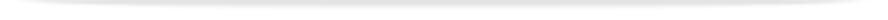units

MAT1841

Faculty of Information Technologyprint version

This unit entry is for students who completed this unit in 2016 only. For students planning to study the unit, please refer to the unit indexes in the the current edition of the Handbook. If you have any queries contact the managing faculty for your course or area of study.

# MAT1841 - Continuous mathematics for computer science

## 6 points, SCA Band 2, 0.125 EFTSL

Refer to the specific census and withdrawal dates for the semester(s) in which this unit is offered.

Faculty

Information Technology

Offered

Clayton

• Second semester 2016 (Day)

Malaysia

• Second semester 2016 (Day)

### Synopsis

The unit covers linear algebra (vectors, equations of lines and planes, solutions of simultaneous equations, determinates, Gauss elimination, Gauss-Jordan method), calculus topics (differentiation, parametric differentiation, fundamental theorem of calculus, and numerical integration), an introduction to multivariable calculus (functions of several variables, partial derivatives, tangent planes and directional derivatives), and the construction of splines and Taylor series expansions are also covered.

### Outcomes

At the completion of this unit, students should be able to:

1. demonstrate basic knowledge and skills of linear algebra, including the manipulation of matrices, solution of linear systems, and evaluate and apply determinants;
2. explain fundamental concepts in calculus including basic differentiation and integration, and composite, inverse and parametric functions;
3. calculate approximations of functions with tangent lines, evaluate power series and construct Taylor series;
4. perform key skills in the calculus of functions of several variables including the calculation of partial derivatives, find tangent planes and identify stationary points.

### Assessment

Examination (3 hours): 70%; In-semester assessment: 30%

Minimum total expected workload equals 12 hours per week comprising:

(a.) Contact hours for on-campus students:

• Three hours lectures
• 1.5 hours laboratories

A minimum of 7.5 hours independent study per week for completing lab and project work, private study and revision.